Open In App
Related Articles
• Write an Interview Experience
• Computer Network Tutorial

# Unicast Routing – Link State Routing

Prerequisite: Distance Vector Routing, Dijkstra algorithm

Unicast means the transmission from a single sender to a single receiver. It is a point-to-point communication between the sender and receiver. There are various unicast protocols such as TCP, HTTP, etc.

• TCP is the most commonly used unicast protocol. It is a connection-oriented protocol that relies on acknowledgment from the receiver side.
• HTTP stands for HyperText Transfer Protocol. It is an object-oriented protocol for communication.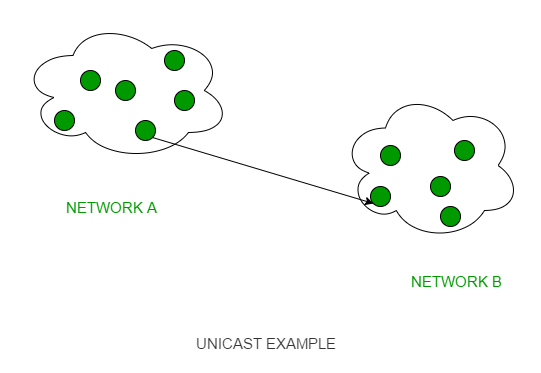Unicast Routing

## Major Protocols of Unicast Routing

1. Distance Vector Routing: Distance-Vector routers use a distributed algorithm to compute their routing tables.
2. Link-State Routing: Link-State routing uses link-state routers to exchange messages that allow each router to learn the entire network topology.
3. Path-Vector Routing: It is a routing protocol that maintains the path that is updated dynamically.

Link state routing is the second family of routing protocols. While distance-vector routers use a distributed algorithm to compute their routing tables, link-state routing uses link-state routers to exchange messages that allow each router to learn the entire network topology. Based on this learned topology, each router is then able to compute its routing table by using the shortest path computation.

Link state routing is a technique in which each router shares the knowledge of its neighborhood with every other router i.e. the internet work. The three keys to understand the link state routing algorithm.

1. Knowledge about the neighborhood: Instead of sending its routing table, a router sends the information about its neighborhood only. A router broadcast its identities and cost of the directly attached links to other routers.
2. Flooding: Each router sends the information to every other router on the internetwork except its neighbors. This process is known as flooding. Every router that receives the packet sends the copies to all the neighbors. Finally each and every router receives a copy of the same information.
3. Information Sharing: A router send the information to every other router only when the change occurs in the information.

Link state routing has two phase:

1. Reliable Flooding: Initial state– Each node knows the cost of its neighbors. Final state- Each node knows the entire graph.
2. Route Calculation: Each node uses Dijkstra’ s algorithm on the graph to calculate the optimal routes to all nodes. The link state routing algorithm is also known as Dijkstra’s algorithm which is used to find the shortest path from one node to every other node in the network.

## Features of Link State Routing Protocols

• Link State Packet: A small packet that contains routing information.
• Link-State Database: A collection of information gathered from the link-state packet.
• Shortest Path First Algorithm (Dijkstra algorithm): A calculation performed on the database results in the shortest path
• Routing Table: A list of known paths and interfaces.

## Calculation of Shortest Path

To find the shortest path, each node needs to run the famous Dijkstra algorithm. Let us understand how can we find the shortest path using an example.

### Illustration

To understand the Dijkstra Algorithm, let’s take a graph and find the shortest path from the source to all nodes.

Note: We use a boolean array sptSet[] to represent the set of vertices included in SPT. If a value sptSet[v] is true, then vertex v is included in SPT, otherwise not. Array dist[] is used to store the shortest distance values of all vertices.

Consider the below graph and src = 0.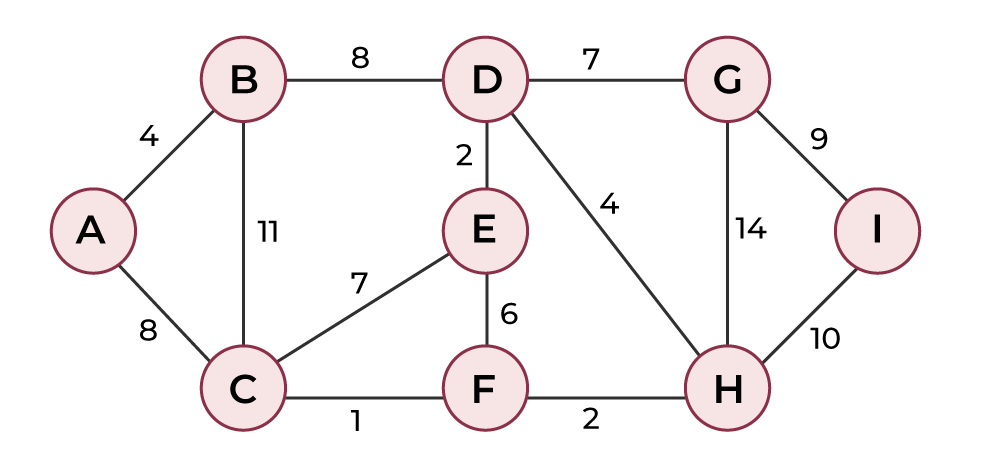Shortest Path Calculation – Step 1

STEP 1: The set sptSet is initially empty and distances assigned to vertices are {0, INF, INF, INF, INF, INF, INF, INF} where INF indicates infinite. Now pick the vertex with a minimum distance value. The vertex 0 is picked and included in sptSet. So sptSet becomes {0}. After including 0 to sptSet, update the distance values of its adjacent vertices. Adjacent vertices of 0 are 1 and 7. The distance values of 1 and 7 are updated as 4 and 8.

The following subgraph shows vertices and their distance values. Vertices included in SPT are included in GREEN color.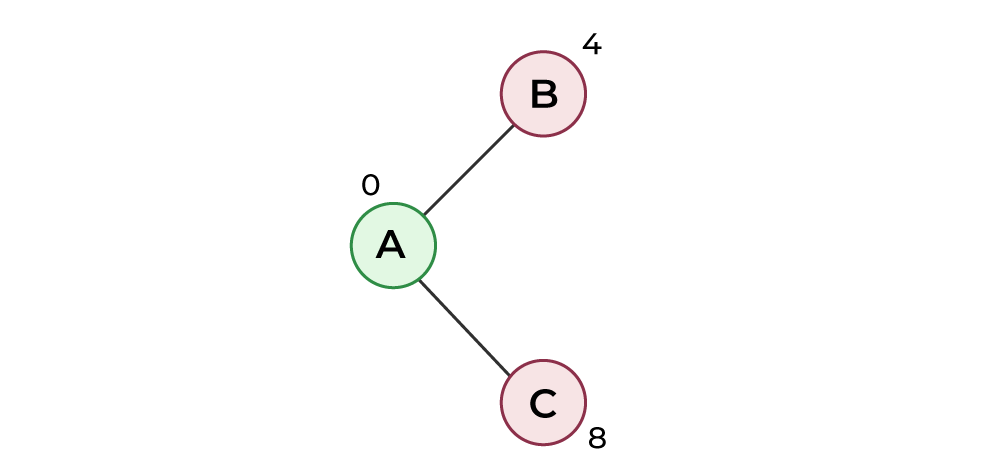Shortest Path Calculation – Step 2

STEP 2: Pick the vertex with minimum distance value and not already included in SPT (not in sptSET). The vertex 1 is picked and added to sptSet. So sptSet now becomes {0, 1}. Update the distance values of adjacent vertices of 1. The distance value of vertex 2 becomes 12.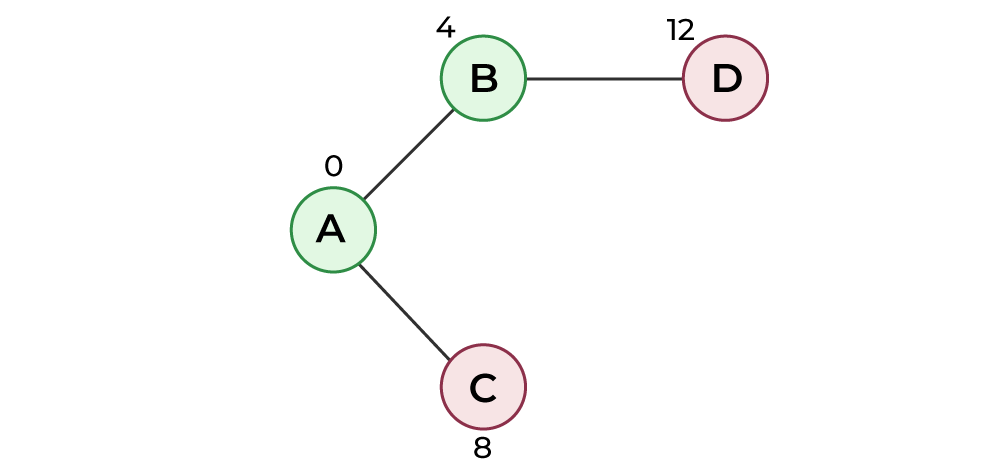Shortest Path Calculation – Step 3

STEP 3: Pick the vertex with minimum distance value and not already included in SPT (not in sptSET). Vertex 7 is picked. So sptSet now becomes {0, 1, 7}. Update the distance values of adjacent vertices of 7. The distance value of vertex 6 and 8 becomes finite (15 and 9 respectively).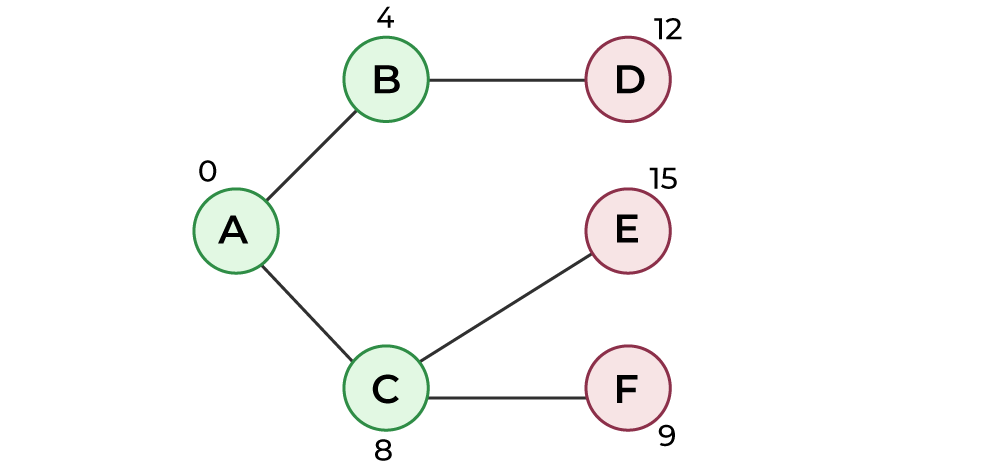Shortest Path Calculation – Step 4

STEP 4: Pick the vertex with minimum distance value and not already included in SPT (not in sptSET). Vertex 6 is picked. So sptSet now becomes {0, 1, 7, 6}. Update the distance values of adjacent vertices of 6. The distance value of vertex 5 and 8 are updated.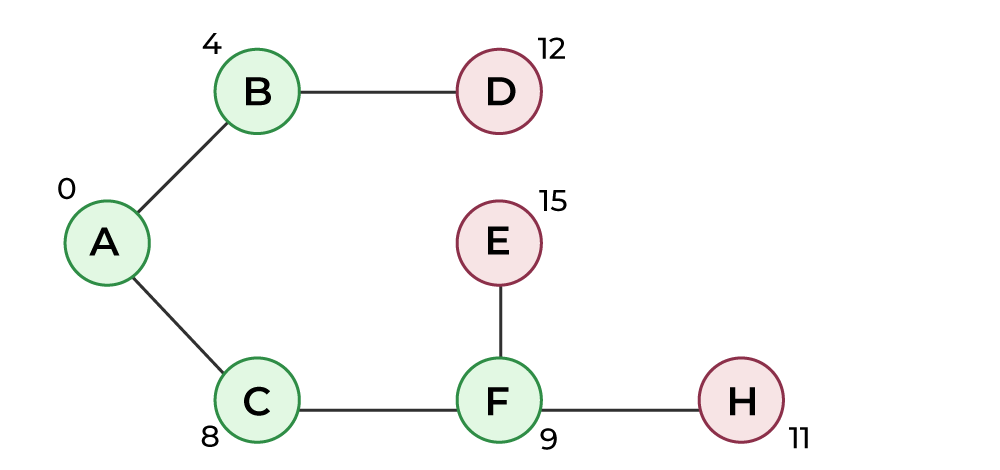Shortest Path Calculation – Step 5

We repeat the above steps until sptSet includes all vertices of the given graph. Finally, we get the following Shortest Path Tree (SPT).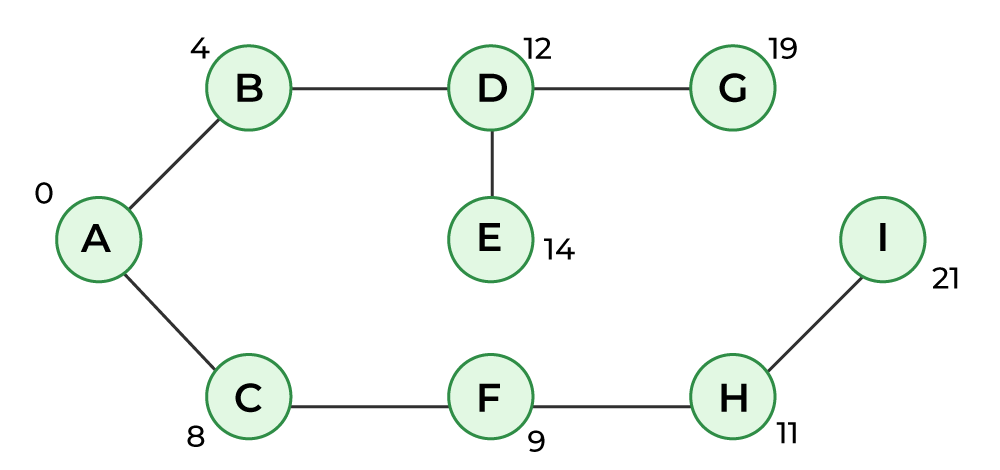Shortest Path Calculation – Step 6

## Characteristics of Link State Protocol

• It requires a large amount of memory.
• Shortest path computations require many CPU circles.
• If a network uses little bandwidth; it quickly reacts to topology changes
• All items in the database must be sent to neighbors to form link-state packets.
• All neighbors must be trusted in the topology.
• Authentication mechanisms can be used to avoid undesired adjacency and problems.
• No split horizon techniques are possible in the link-state routing.
• OSPF Protocol

## Protocols of Link State Routing

1. Open Shortest Path First (OSPF)
2. Intermediate System to Intermediate System (IS-IS)

Open Shortest Path First (OSPF): Open Shortest Path First (OSPF) is a unicast routing protocol developed by a working group of the Internet Engineering Task Force (IETF). It is an intradomain routing protocol. It is an open-source protocol. It is similar to Routing Information Protocol (RIP). OSPF is a classless routing protocol, which means that in its updates, it includes the subnet of each route it knows about, thus, enabling variable-length subnet masks. With variable-length subnet masks, an IP network can be broken into many subnets of various sizes. This provides network administrators with extra network configuration flexibility. These updates are multicasts at specific addresses (224.0.0.5 and 224.0.0.6). OSPF is implemented as a program in the network layer using the services provided by the Internet Protocol. IP datagram that carries the messages from OSPF sets the value of the protocol field to 89. OSPF is based on the SPF algorithm, which sometimes is referred to as the Dijkstra algorithm.

Intermediate System to Intermediate System (IS-IS): Intermediate System to Intermediate System is a standardized link-state protocol that was developed as the definitive routing protocol for the OSI Model. IS-IS uses System ID to identify a router on the network. IS-IS doesn’t require IP connectivity between the routers as updates are sent via CLNS instead of IP.

For more about OSPF and IS-IS, you can refer Difference between OSPF and IS-IS article.